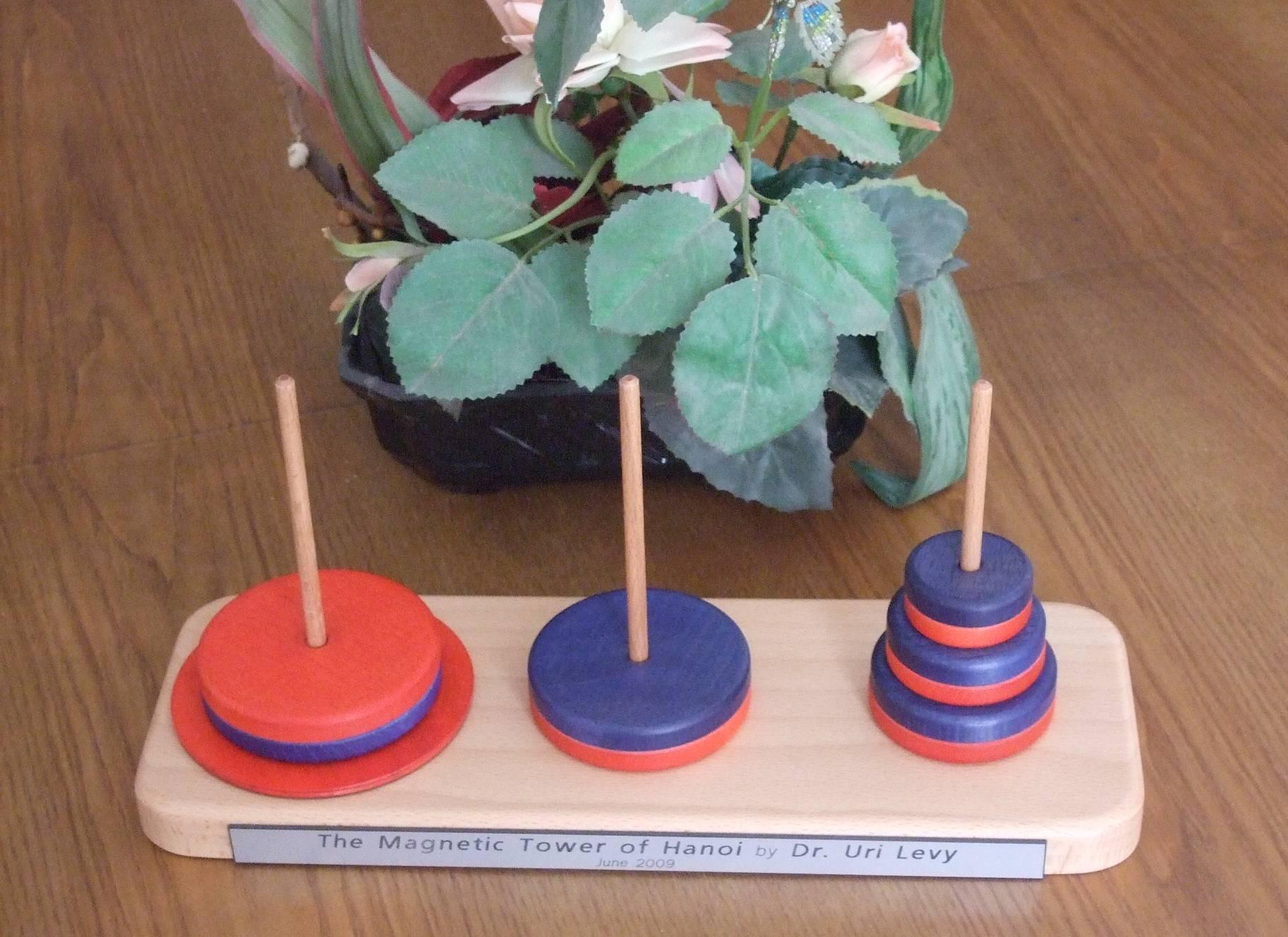# The Magnetic Tower of Hanoi

 Page 17/20 Date 23.04.2018 Size 2.78 Mb. #45758
MToH puzzle-solving algorithms presented in this paper. Yet, before concluding, I wish to bring forward another section. A "Color-Crossings" section that is. The section presents the color of each of the three posts during the entire solving procedure, in a graphical form. Shedding colorful light onto the MToH puzzle.

Let's see.

1. Color-Crossings

To visualize color-crossings, I asked the computer to record the color of each post for each move, from start to finish, and designate each color by a number - "1" for Red, "0" for Neutral and "-1" for Blue.

Selected recordings are shown by the two figures below.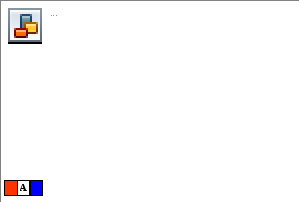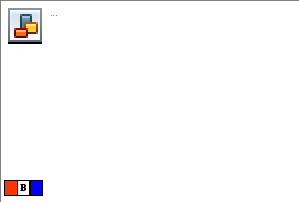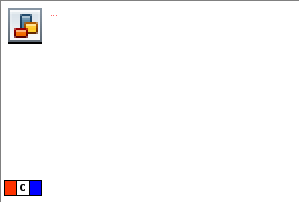Figure 13: Color-Crossings charts. All three charts are associated with a height 3 MToH. A – Colored MToH and the "100" Algorithm. 13 moves, No Color-Crossing. B – Free MToH and the "100" Algorithm. Still 13 moves. Still no Color-Crossing (see text for details). C - Free MToH and the "67-Down" Algorithm. Two Color-Crossings, only 11 moves.

The three charts of Figure 13 all relate to height 3 of the MToH.

The top one (A) shows the color of each of the posts for a Colored MToH (CMToH). In this case of a "Permanently Colored" Tower, the posts are pre-colored Red-Blue-Blue and the "100" Algorithm curves, not surprising, stay horizontal throughout the entire 13-move solution.

The middle one (B) relates to a "regular" or "Free" MToH, solved by exactly the same "100" Algoritm as was the case for 13A. Now, during the 13-move solution, we see each of the three posts wonders between Neutral and one color, never crossing Neutral to "visit" the opposite color.

The bottom one (C) relates to the "62-Down" Algorithm, solving the MToH puzzle in, as we know very well by now, just 11 moves. In this case we see two Color-Crossings. By "Color-Crossing" I refer to a move sequence where a post goes from one color through Neutral (and may stay there for a short while) to the opposite color. Such Color-Crossing is exersiced by the Intermediade Post in moves 3,4 and 5 and by the Destination Post in moves 7,8 and 9 of the "62-Down" Algorithm. These Color-Crossings "take responsibility" for the shorter-duration solution of only 11 moves.

Next, Figure 14 shows Color-Crossing charts for an MToH of height 5, comparing the crossings of the "67-Down" Algorithm (A) to the crossings of the "62" Algoritm (B).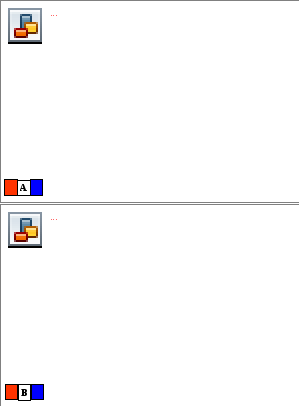Figure 14: Color-Crossings charts for a Free MToH of height 5. A – "67-Down" Algorithm. Six Color-Crossings (see Table 10 below). 85 moves. B – "62" Algorithm. Eight Color-Crossings (see Table 10 below). Eighty-three moves.

Comparing the top chart (Figure 5A) to the bottom chart (Figure 5B), We see additional two Color-Crossings of the Source-Post (71 through 73 ; 74 through 76) for the "62" Algorithm (Figure 5B). Again we wittness the correlation between larger number of Color-Crossings and a solution of a shorter duration.

To see this Crossings-Duration correlation, we listed in Table 10 the number of Color-Crossings of each post for the first eight MToH heights.

 N 1 2 3 4 5 6 7 8 67-Down-S 0 0 0 0 0 0 0 0 67-Down-I 0 0 1 2 3 4 5 6 67-Down-D 0 0 1 2 3 4 5 6 67-Down-total 0 0 2 4 6 8 10 12 P67-Down(N) 1 4 11 30 85 248 735 2194 67-Up-S 0 0 0 2 2 4 4 6 67-Up-I 0 0 1 1 3 3 5 5 67-Up-D 0 0 0 0 0 0 0 0 67-Up-total 0 0 1 3 5 7 9 11 P67-Up(N) 1 4 11 30 85 248 735 2194 62-S 0 0 0 1 2 2 4 4 62-I 0 0 1 2 3 8 9 14 62-D 0 0 0 2 3 4 5 6 62-total 0 0 1 5 8 14 18 24 P62(N) 1 4 11 30 83 236 691 2050

Table 10: Color-Crossings for three algorithms for the first eight MToH heights. For "high" Towers, the posts in the "62" Algorithm make significantly larger numbers of Color-Crossings vs. the corresponding numbers for the two "67" Algorithms.
We did that for three Algorithms – "67-Down", "67-Up" and "62". We had to split the "67" Algorithm because, as shown, the Color-Crossing pattern for the "67-Down" Algorithm differs slightly from the Color-Crossing pattern for the "67-Up" Algorithm. Both, however, solve the MToH puzzle in exactly the same number of moves. And while both are characterized, for each stack height, by a similar number of crossings, they both display significantly smaller number of Color-Crossings (for "high" stacks) when compared to the number of Color-Crossimgs of the "62" Algorithm. And we know that the "62" Algorithm solution is of shorter duration. For high stacks then, the correlation discovered and discussed in relation to height 3, and height 5 persists.
So much for the MToH move analysis.

Now just a few organizing remarks before concluding.

All four Algorithms discussed above – "100", "67", "SemiFree" and "62", are recursive. Explicit recursive functions that run on NUMERIT ("Mathematical & Scientific Computing") are listed in Appendix 1. Also listed in Appendix 1 are "program managing" functions that were written for program clarity and for better program managability.

A "movie" showing the "62" Algorithm solving a height five MToH in (only) 83 moves can be seen here.

A few pictures, showing actual realization of the MToH puzzle, are shown in Appendix 2.

Let's conclude now.

1. Concluding remarks

The task of the "Monks of Hanoi" is nearing completion. The big disk has been moved. Evidently, 263 = 9.223372036854775808*1018 seconds have already past since the Monks started performing their routine (always without the slightest hesitation). So SOON "the world will end" ! If only the command of the ancient prophecy would have been to move the 64 disks under the rules of the Magnetic Tower of Hanoi. If that was the case, we would still have

((364-1)/2)*(67/108) – 263 = 3.550259505549357568*1029 seconds of colorful life ahead of us (out of the original 1.06507785166480704*1030 seconds since they started). But let's not worry. Let's enjoy our world, with the innovations it offers, for the remaining 9.223372036854775808*1018 seconds.

"Always without the slightest hesitation". I used this phrase in the previous paragraph. Because as a matter of fact, for the classical base-2 ToH, determinism prevails. If the play moves "forward" (on the down-sequence for example, N-2 disks go over the freshly moved disk number N-1 and not back over disk number N) then the moves are mandatory. No need to think, no reason to hesitate. The same applies to the Colored Magnetic Tower of Hanoi. True, both Towers span their respective bases perfectly, but the puzzle solution has an element of monotony in it. Solving (efficiently) the Free Magnetic Tower of Hanoi puzzle is a different story. On one hand, when counting moves, the number "3" stars. If you look back through this paper, you will find this number (3) in all of the equations from Equation 3 and on. Without exception. In some early equations implicitely. These early "hints" do not decieve us. As we easily realize now - "1" is actually 30 ; "4" is actually (32 - 30)/(31 - 30) ; "11" is actually 3(3 - 1) + (3 - 1). And so, indeed, number "3" is everywhere. However, not only number 3 stars, but the game is intricate. The puzzle solution may progess in more than one path. The puzzle presents more than one option to the player. The Tower therefore calls for thinking, justifies hesitation. It is Freedom that makes the MToH puzzle so colorful.

1. References

 http://en.wikipedia.org/wiki/%C3%89douard_Lucas

 http://en.wikipedia.org/wiki/Tower_of_Hanoi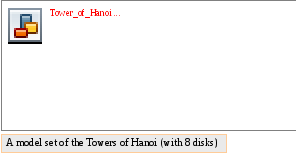If the legend were true, and if the priests were able to move disks at a rate of one per second, using the smallest number of moves, it would take them 264−1 seconds or roughly 600 billion years; it would take 18,446,744,073,709,551,615 turns to finish.

 http://www.cut-the-knot.org/recurrence/hanoi.shtml
 "The Magnetic Tower of Hanoi", Uri Levy, Journal of Recreational Mathematics 35:3, to be published (~April 2010)
 http://www.numerit.com/
 A "movie" showing the "62" Algorithm solving a height five MToH in (only) 83 moves:
http://www.numerit.com/maghanoi/

User name: maghanoi

Appendix 1: Recursive functions for the "62" solution
Listed in this Appendix are all the functions by which the "62" Algorithm solves the MToH puzzle. The functions run on NUMERIT – a "Mathematical & Scientific Computing" environment. Five of the functions (D,G,H,I,J) are recursive (call themselves). These functions, the "heart" of the game, may offer important clues needed to decipher the MToH puzzle.

solve_MToH_puzzle(n,s,d,i)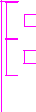function solve_MToH_puzzle(n,s,d,i)

if n=1

move_down_67(n,s,d,i)

return

if n=2

move_down_67(n,s,d,i)

return

move_down_67(n-1,s,i,d)

move(n,s,d)

move_all_but_n_up(n,i,d,s)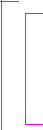function move_all_but_n_up(n,i,d,s)

if n > 2

move_busy_2and1(n-2,i,s,d)

move_busy_1and2(n-2,s,d,i)

move(n-1,i,s)

move_semifree_BNR(n-3,d,s,i)

move(n-2,d,i)

move_busy_2and1(n-3,s,d,i)

move_busy_1and2(n-3,d,i,s)

move(n-1,s,d)

move_up_67(n-2,i,d,s)

function move_semifree_BNR(n,s,d,i)

if n > 2

move_semifree_BNR(n-2,s,d,i)

move(n-1,s,i)

move_busy_2and1(n-2,d,s,i)

move_busy_1and2(n-2,s,i,d)

move(n,s,d)

move_busy_2and1(n-2,i,s,d)

move_busy_1and2(n-2,s,d,i)

move(n-1,i,s)

move_busy_1and2(n-2,d,i,s)

move(n-1,s,d)

move_busy_2and1(n-2,i,d,s)

return

if n = 1

move_busy_2and1(1,s,d,i)

return

if n = 2

move_busy_2and1(2,s,d,i)

return

function move_down_67_3disks(n,s,d,i)

if n > 0

move_busy_2and1(n-1,s,i,d)

move(n,s,d)

move_busy_1and2(n-2,i,s,d)

move_busy_2and1(n-2,s,d,i)

move(n-1,i,s)

move_busy_2and1(n-2,d,i,s)

move(n-1,s,d)

move_busy_1and2(n-2,i,d,s)

function move_up_67_3disks(n,s,d,i)

if n > 0

move_busy_2and1(n-2,s,i,d)

move(n-1,s,d)

move_busy_1and2(n-2,i,s,d)

move(n-1,d,i)

move_busy_1and2(n-2,s,d,i)

move_busy_2and1(n-2,d,i,s)

move(n,s,d)

move_busy_2and1(n-1,i,d,s)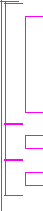function move_down_67(n,s,d,i)

if n > 3

move_down_67(n-1,s,i,d)

move(n,s,d)

move_busy_2and1(n-2,i,s,d)

move_busy_1and2(n-2,s,d,i)

move(n-1,i,s)

move_busy_1and2(n-2,d,i,s)

move(n-1,s,d)

move_busy_2and1(n-2,i,d,s)

return

if n=2

move_busy_1and2(2,s,d,i)

return

if n=1

move_busy_1and2(1,s,d,i)

return

move_down_67_3disks(3,s,d,i)

function move_up_67(n,s,d,i)if n > 3

move_busy_1and2(n-2,s,i,d)

move(n-1,s,d)

move_busy_2and1(n-2,i,s,d)

move(n-1,d,i)

move_busy_2and1(n-2,s,d,i)

move_busy_1and2(n-2,d,i,s)

move(n,s,d)

move_up_67(n-1,i,d,s)

return

if n=2

move_busy_1and2(2,s,d,i)

return

if n=1

move_busy_1and2(1,s,d,i)

return

move_up_67_3disks(3,s,d,i)

function move_busy_2and1(n,i,s,d)

if n > 0

move_busy_2and1(n-1,i,s,d)

move_busy_1and2(n-1,s,d,i)

move(n,i,s)

move_busy_2and1(n-1,d,s,i)

function move_busy_1and2(n,s,d,i)

if n > 0

move_busy_1and2(n-1,s,i,d)

move(n,s,d)

move_busy_2and1(n-1,i,s,d)

move_busy_1and2(n-1,s,d,i)

Appendix 2: Realization of the Magnetic Tower of Hanoi

Below – a few pictures of a realized version of the Magnetic Tower of Hanoi.